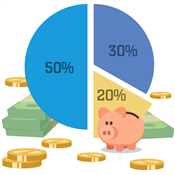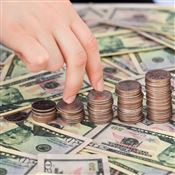# Discount Calculator

How do you calculate discounts? Use these free calculators to see how much you'll pay before and after discounts are applied to your next purchase.Shopping can sometimes feel like a math quiz.

When discounts are applied, how much are you really paying?

## How do I calculate a discount percentage?

Discount Calculator

To calculate the discount percentage taken off your item, follow these steps:

1. Find the original price of the item before the discount.
2. Find the price of the item after the discount was applied.
3. Subtract the after-discount price from the original price.
4. Divide that by the original price.
5. Multiply by 100.
6. This number is the percentage that was deducted from the item price.

Calculate discount percentage example
\$125 (original price) - \$100 (after-discount price) = \$25
25 / \$125 = 0.2
0.2 x \$100 = 20
Discount percentage = 20%

## How to find the original price before a discount

Price Before Discount Calculator

To calculate the original price of an item before the discount is applied, follow these steps:

1. Find the price of the item after the discount was applied.
2. Find the discount percentage that was applied to the price.
3. Divide the discount percentage by 100.
4. Subtract that number from 1.
5. Divide the after-discount price by that number.
6. This number is the original price of the item, pre-discount.

Calculate price before discount example
20 (discount percentage)/ 100 = 0.2
1 - 0.2 = 0.8
\$100 / 0.8 = 125
Price before discount = \$125

## How to find the price after a discount

Price After Discount Calculator

To calculate the price of an item after the discount is applied, follow these steps:

1. Find the original price of the item.
2. Find the discount percentage that will be applied to the price.
3. Divide the original price by 100.
4. Multiply by the discount percentage.
5. Subtract that number from the original price.
6. This number is the discounted price.

Calculate price after discount example
\$125 (original price) / 100 = 1.25
1.25 x 20 (discount percentage) = 25
\$125 - 25 = \$100
Price after discount = \$100

## How do you calculate a 5% discount?

1. Find the original price of the item.
2. Divide the original price by 100.
3. Then, multiply by 5.
4. Subtract that number from the original price.
5. The new number is the discounted price.

5% Discount Example
\$200 (original price) / 100 = 2
2 x 5 = 10
200 - 10 = 190
Discounted price: \$190

Looking for more ways to save on your everyday purchases? Check out our guide to the best cash back apps to learn how to put more of your hard-earned cash back in your pocket.

## How do you calculate a 10% discount?

1. Find the original price of the item.
2. Divide the original price by 100.
3. Then, multiply by 10.
4. Subtract that number from the original price.
5. The new number is the discounted price.

10% Discount Example
\$200 (original price) / 100 = 2
2 x 10 = 20
200 - 20 = 180
Discounted price: \$180

## How do you calculate a 20% discount?

1. Find the original price of the item.
2. Divide the original price by 100.
3. Then, multiply by 20.
4. Subtract that number from the original price.
5. The new number is the discounted price.

20% Discount Example
\$200 (original price) / 100 = 2
2 x 20 = 40
200 - 40 = 160
Discounted price: \$160

## How do you calculate a 25% discount?

1. Find the original price of the item.
2. Divide the original price by 100.
3. Then, multiply by 25.
4. Subtract that number from the original price.
5. The new number is the discounted price.

25% Discount Example
\$200 (original price) / 100 = 2
2 x 25 = 50
200 - 50 = 150
Discounted price: \$150

## What are the types of discounts?

Percentage discount: This is when a percentage (between 1 - 100) is deducted from the item's price (for example, 10% off \$50 = \$45)

Flat amount discount: This is when a flat dollar amount is deducted from the item's price (for example \$5 off \$50 = \$45)

"Buy one, get one" discount: This is when you buy one item at full price and get a second item discounted (or even free!)

Quantity discount: This is when the retailer gives you a discount for buying multiple items

Trade discount: This is a discount usually offered by a wholesaler to a retailer (for example, a flower wholesaler giving a discount to a florist who sells their flowers)

Discounts aren't the only thing that influence how much you pay. Find out how sales tax affects your purchase with our handy sales tax calculators.

### Bottom Line

Before buying something, it pays to shop around and find the best deal. But even if you're getting a discount, don't go in unprepared.

With these calculators, you'll know exactly how much you're being charged so you can shop with confidence.

Holly Zorbas is a assistant editor at CreditDonkey, a credit card comparison and reviews website. Write to Holly Zorbas at holly.zorbas@creditdonkey.com. Follow us on Twitter and Facebook for our latest posts.#### Sales Tax Calculator### Best Cash Back Apps### How to Make a Budget### How Much Should I Save?#### Is a Savings Account Worth It### Online Budgeting Tools#### Hostgator Review#### Chase Ink Business Unlimited Review#### NorthOne Review